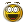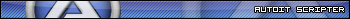Recommended Posts

Hello, I am new to AutoIt and I am wondering something. Is it possible to enter a calculation (such as 5*3) in a single inputbox and calculate it?

For example:

\$Calculation = InputBox( "Calculator", "Enter Your Calculation Here:", "", " M")

So basically I am asking if it is possible to get \$Calculation as one number, or do something with it to make it one number (so if I would enter 3*5 it would change it into 15), in another variable of course.

~Ardel1

Share on other sites

of course yes.

\$Calculation = InputBox( "Calculator", "Enter Your Calculation Here:", "", " M")
MsgBox(0,"",Execute(\$Calculation))

SaludosDanysys.comAutoIt...

Share on other sites

Thank you very much!

I honestly expected it would be something that simple but I just couldn't find it.

Thanks again :)!